# The sum

The sum of the first 10 members of the arithmetic sequence is 120. What will be the sum if the difference is reduced by 2?

s2 =  30

### Step-by-step explanation: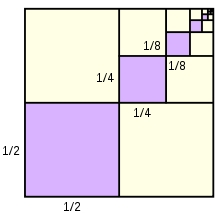Did you find an error or inaccuracy? Feel free to write us. Thank you!Tips to related online calculators
Looking for help with calculating arithmetic mean?
Looking for a statistical calculator?

#### You need to know the following knowledge to solve this word math problem:

We encourage you to watch this tutorial video on this math problem:

## Related math problems and questions:

• Finite arithmetic sequenceHow many numbers should be inserted between the numbers 1 and 25 so that all numbers create a finite arithmetic sequence and that the sum of all members of this group is 117?
• Difference AP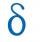Calculate the difference of arithmetic progression if the sum of its first 19 members Sn = 8075 and the first member is a1 = 20
• SequenceBetween numbers 1 and 53 insert n members of the arithmetic sequence that its sum is 702.
• AS - sequence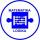What are the first ten members of the sequence if a11=22, d=2.
• AS sequenceIn an arithmetic sequence is given the difference d = -3 and a71 = 455. a) Determine the value of a62 b) Determine the sum of 71 members.
• AP - simpleFind the first ten members of the sequence if a11 = 132, d = 3.
• GP membersThe geometric sequence has 10 members. The last two members are 2 and -1. Which member is -1/16?
• No. 215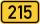From the number 215 we can create a four-digit number that among its numbers manually type any other digit. Thus, we created two four-digit numbers whose difference is 120. What two four-digit numbers that might be?
• Find the sumFind the sum of all natural numbers from 1 and 100, which are divisible by 2 or 5
• Sum of membersWhat is the sum of the first two members of the aritmetic progression if d = -4.3 and a3 = 7.5?
• Three unknown numbers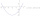The sum of of three numbers is 106. the first number is 10 more then the third. The second number is 2x the third. What are the numbers?
• Statistics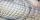The sum of all deviations from the arithmetic mean of the numerical sequence 4, 6, 51, 77, 90, 93, 95, 109, 113, 117 is:
• In the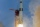In the arithmetic sequence a1 = 4.8, d = 0.4. How many consecutive members, starting with the first, need to be added so that the sum is greater than 170?
• Geometric sequence 4It is given geometric sequence a3 = 7 and a12 = 3. Calculate s23 (= sum of the first 23 members of the sequence).
• Loan 5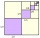Abdul takes a loan of 200000 from Ali and agrees to repay in number of instalment, each instalment begin with the 2nd exceeding the previous one by 1000, if the first instalment is 500, find how many instalment will be necessary to be wipe out the loan? C
• Five element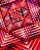The geometric sequence is given by quotient q = 1/2 and the sum of the first six members S6 = 63. Find the fifth element a5.
• Three members GPThe sum of three numbers in GP (geometric progression) is 21 and the sum of their squares is 189. Find the numbers.